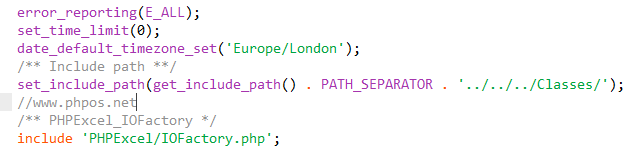028 - 61833149

# DEDE批量导入excel数据到后台文章系统的开发教程

1）开发前的准备：2) 开发插入数据代码：

`<?phpheader("Content-type:text/html;charset=utf8");require_once('/../dedecms/include/common.inc.php'); error_reporting(E_ALL);set_time_limit(0);date_default_timezone_set('Europe/London');/** Include path **/set_include_path(get_include_path() . PATH_SEPARATOR . '../../../Classes/');/** PHPExcel_IOFactory */include 'PHPExcel/IOFactory.php';if(!empty(\$_GET)){    \$typeid = \$_GET['typeid'];    \$dopost = \$_GET['do'];    if(\$dopost == "exdata"){            if(!empty(\$_GET['n'])){                \$inputFileName = './'.\$_GET['n'].'.xlsx';                \$objPHPExcel = PHPExcel_IOFactory::load(\$inputFileName);                \$sheetData = https://www.jb51.net/cms/\$objPHPExcel->getActiveSheet()->toArray(null,true,true,true);                \$rowarr=array();                \$dsql->Execute('all',"select bio2,bio1 from `dede_addonshop`");                while (\$rowall = \$dsql->GetArray('all')) {                      \$rowarr[]=\$rowall;                }foreach (\$sheetData as \$v) {    foreach (\$rowarr as \$vt) {        if(in_array(\$v['B'],\$vt)&&\$v['A']==\$vt['bio1']){            echo "货号为：".\$v['B']."<br>厂商为：".\$v['A']."<br>的数据已在表中，请在excel文件里面删除这一条后再添加！";            exit;        }    }}                //附加表插入数据前处理                \$row = \$dsql->GetOne("select aid,bio2 from `dede_addonshop` order by aid desc");                if(!empty(\$row)){                    \$aid = \$row['aid'];                    \$bio2 = \$row['bio2'];                }else{                    \$aid = 0;                    \$bio2 = '';                }                //处理重复问题                 if(\$bio2==\$sheetData[count(\$sheetData)]['B']){                  ShowMsg("不能重复添加内容",'javascript:;');                  exit;                  }               //主表插入数据前处理                \$arcrow = \$dsql->GetOne("select id from `dede_archives` order by id desc");                if(!empty(\$arcrow)){                    \$arcid = \$arcrow['id'];                }else{                    \$arcid = 0;                }               //微表插入数据前处理                \$tinyrow = \$dsql->GetOne("select id from `dede_arctiny` order by id desc");                if(!empty(\$tinyrow)){                    \$tinyid = \$tinyrow['id'];                }else{                    \$tinyid = 0;                }                //找出最大的id               \$id = max(\$aid,\$arcid,\$tinyid);               \$alphalpha = array('A','B','C','D','E','F','G','H','I','J','K','L','M','N','O','P','Q','R','S','T','U','V','W','X','Y','Z','AA','AB','AC','AD','AE','AF','AG','AH','AI','AJ','AK','AL','AM','AN','AO','AP','AQ','AR','AS','AT','AU','AV','AW','AX','AY','AZ');               //字段数量               \$fieldnum = count(\$sheetData);               \$fields = \$fieldvaluehttps://www.jb51.net/cms/= '';               for (\$i=0; \$i < \$fieldnum; \$i++) {                      \$fields .= \$sheetData[\$alphalpha[\$i]]. ',';                 }               \$fields = substr(\$fields, 0,-1);              //遍历数组              foreach (\$sheetData as \$value) {                   \$pubdate = GetMkTime(GetDateTimeMk(time()));                   \$click = mt_rand(50, 200);                     if(\$value['A']=='bio1'|\$value['A']=='厂商'){                                continue;                            }                      \$id = \$id+1;                       //获取字段值\$value['A'];                        for (\$i=0; \$i < \$fieldnum; \$i++) {                               \$fieldvalue .= " ,'".\$value[\$alphalpha[\$i]]."' ";                         }                     //标题                     \$C = trim(\$value['C']);                    //保存到主表                     \$senddate = time();                     \$arcquery = "INSERT INTO `dede_archives`(id,typeid,title,mid,channel,pubdate,senddate,click,ismake)VALUES ('\$id','\$typeid','\$C','1','6','\$pubdate','\$senddate','\$click','-1');";                      \$dsql->ExecuteNoneQuery(\$arcquery);                    //保存到附近加表                    \$query = "INSERT INTO `dede_addonshop`(aid,typeid,\$fields)                    VALUES ('\$id','\$typeid'{\$fieldvalue});";                    \$dsql->ExecuteNoneQuery(\$query);                    \$fieldvaluehttps://www.jb51.net/cms/= '';                    //保存到微表                    \$tinyquery = "INSERT INTO `dede_arctiny`(id,typeid,channel,mid,senddate)VALUES ('\$id','\$typeid','6','1','\$senddate');";                    \$dsql->ExecuteNoneQuery(\$tinyquery);                 }        \$num = count(\$sheetData)-2;        ShowMsg("恭喜，成功插入   ".\$num."   条数据！",'javascript:;');      }    }}else{    echo "密码或文件名错误！您无权做任何操作！";}?>`

1）第一步先判断问号后面是不是为空，即dopost=exdata&typeid=6&n=test1字符串是否为空，如果这个字符串都是空的话，其它，就不用操作了，直接通过出程序，显示：echo "密码或文件名错误！您无权做任何操作！";

`\$typeid = \$_GET['typeid'];\$dopost = \$_GET['do'];`

3）插入数据库表时最大数据id的判断

3）遍历数组\$sheetData插入数据

### 相关新闻

2008-2020 © 成都零起飞科技有限公司 网站建设 开源CRM 建站系统 旅行社ERP系统 虚拟主机